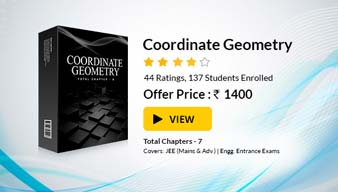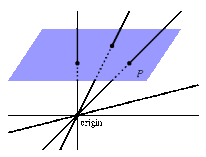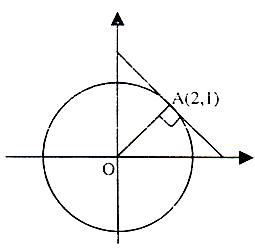×#### Thank you for registering.

One of our academic counsellors will contact you within 1 working day.

Click to Chat

1800-1023-196

+91-120-4616500

CART 0

• 0

MY CART (5)

Use Coupon: CART20 and get 20% off on all online Study Material

ITEM
DETAILS
MRP
DISCOUNT
FINAL PRICE
Total Price: Rs.

There are no items in this cart.
Continue Shopping```Concurrency of Straight Lines

What do you mean by intersection of three lines or concurrency of straight lines?Suppose we have three staright lines whose equations are a1x + b1y + c1 = 0, a2x + b2y + c2 = 0 and a3x + b3y + c3 = 0. These lines are sid to be concurrent if the following condition holds good:= 0.

If we have three straight lines with equations L1 = 0, L2 = 0 and L3 = 0, then they are said to be concurrent if there exist three constants a, b and c not all zero such that aL1 + bL2 + cL3 = 0.

Generally speaking, three lines are said to be concurrent if any one of the lines passes through the point of intersection of the other two lines.Now, we discuss certain illustrations based on this concept:

Illustration:

Check if the following lines are concurrent

a1x + b1y + c1 = 0                                             …… (1)

a2x + b2y + c2 = 0                                             …… (2)

(2a1 – 3a2)x + (2b1 – 3b2)y + (2c1 – 3c2) = 0       …… (3)

Solution:

On carefully seeing the coeffcients we realize that if the lines are denoted by L1, L2 and L3, then we have L3 – 2L1 + 3L2 = 0. Hence, using the above fact, we have found three constants not all zero such that aL1 + bL2 + cL3 = 0. Hence, the lines are concurrent.

Result:

How many lines can pass through the intersection of two lines? Can we find a general equation of these lines?

If L1 = 0 and L2 = 0 are two lines then equation of family of lines passing through their intersection is given by

L1 + λL2 = 0                                                       …… (A)

Where ‘λ’ is any parameter. (Equation A is satisfied by the point of intersection of L1 = 0 and L2 = 0)

Note:

To determine a particular line, one more condition is required so as to determine or eliminate λ .

Watch this Video for more reference

Illustration:

Find the equation of a line passing through the point of intersection of x + y – 3 = 0 and 2x – y + 1 = 0 and a point (2, -3).

Solution:

Any line passing through the point of intersection of the lines x + y – 3 = 0 and 2x – y + 1 = 0 is given by

(x + y – 3) +A(2x – y + 1) = 0

Now, it passes through the point (2, -3) and so (2-3-3) + A(2.2 – (-3) + 1) = 0

This gives 8A – 4 = 0 which gives A = 1/2

Hence, the required equation is (x + y – 3) + 1/2(2x – y + 1) = 0 which is 4x + y – 5 = 0.

Illustration:

Find the equation of a line, through the intersection of 2x + 3y – 7 = 0 and x + 3y – 5 = 0 and having distance from origin as large as possible.Solution:

Point of intersection of two lines is A(2, 1).

Now, with OA as radius and O itself as centre draw a circle.

There will be infinitely many lines through A and each except one of them produces a chord of circle and hence their distance from origin i.e. centre of circle is less than OA i.e. radius of circle.

But the exceptional one which infact is a tangent to circle at A will be at a distance OA from O.

Thus, tangent to circle at A will be the line through A and is farthest from origin.

Now, OA ⊥ tangent at A.

Hence, (slope of OA) × (slope of tangent at A) = –1

Or, (slope of tangent at A) = –2 which means (1–0)/(2–0) = -2.

Hence, equation of required line is (y – 1) = –2(x – 2)

or 2x + y – 5 = 0.

Illustration:

Find the point of concurrency of the altitudes drawn from the vertices (at1t2, a(t1 + t2)), (at2t3, a(t2 + t3)) and (at3t1, a(t3 + t1)) respectively of a triangle ABC.

Solution:

Equation of AD is y – a(t1 + t2) = –t3(x + at1t2).                … (1)

Equation of CF is y – a(t3 + t1) = –t2(x – at3t1).                 … (2)Subtracting (1) from (2), we get

x = –a which gives y = a(t1 + t2 + t1t2t3).

Hence the point of concurrency of the altitudes is

(–a, a(t1 + t2 + t3 + t1t2t3)).

Related Resources

For getting an idea of the type of questions asked, refer the previous year papers.

To read more, Buy study materials of Straight Lines comprising study notes, revision notes, video lectures, previous year solved questions etc. Also browse for more study materials on Mathematics here.
```### Course Features

• 731 Video Lectures
• Revision Notes
• Previous Year Papers
• Mind Map
• Study Planner
• NCERT Solutions
• Discussion Forum
• Test paper with Video Solution